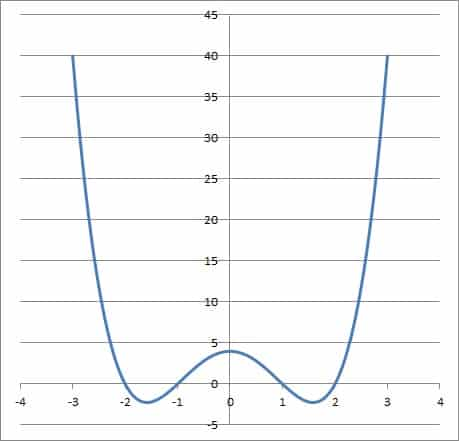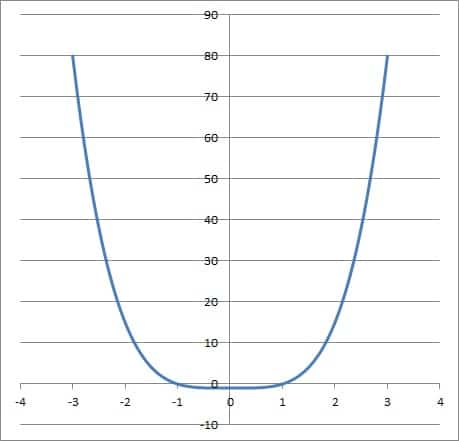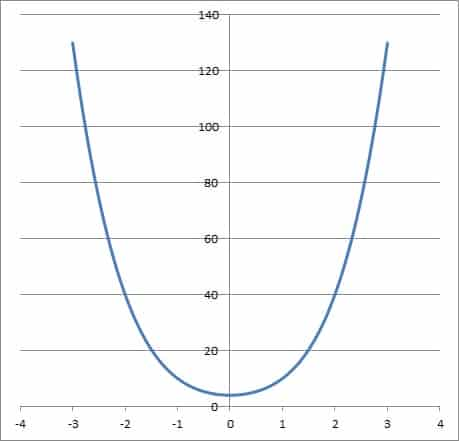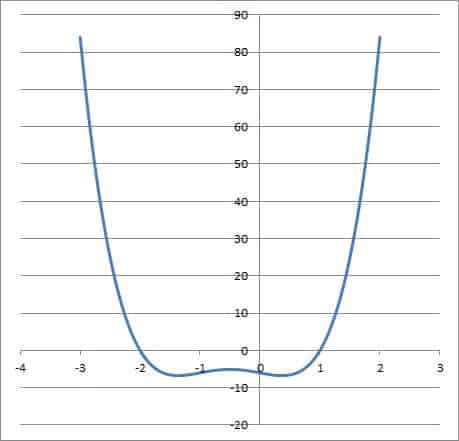# What Is A Quartic Function? (3 Key Things To Know)

Quartic functions are used to find the intersection of two ellipses or the eigenvalues of a 4 by 4 matrix.  However, there is a lot to know about these fourth degree polynomials, their roots, and their graphs.

So, what is a quartic function?  A quartic function has the form f(x) = ax4 + bx3 + cx2 + dx + e, where a, b, c, d, & e are real, with a not zero. A quartic function can have even symmetry, but it cannot be odd or one to one. A quartic function with real coefficients has 4 roots, and it can have 0, 2, or 4 complex/imaginary roots.

Of course, a quartic function can have up to three local extrema (maximums or minimums).  In some cases, a quartic may not have an inflection point (since the second derivative is a quadratic, or second-degree polynomial, some of which will not change signs).

In this article, we’ll take a closer look at quartic functions and answer some common questions about them.  We’ll also look at some examples, including graphs of various quartic functions.

Let’s get started.

## What Is A Quartic Function?

A quartic function is one that has the standard form

• f(x) = ax4 + bx3 + cx2 + dx + e

where a, b, c, d, and e are real, with a not equal to zero.

A quartic function is also called a fourth degree polynomial, or a polynomial function of degree 4.  This means that x4 is the highest power of x that has a nonzero coefficient.

This also means that a quartic polynomial can be described as an even-degree polynomial.  This means that a quartic equation always has an even number of real roots: 0, 2, or 4 (and also an even number of complex/imaginary roots, which come in conjugate pairs).

The domain of a quartic function is the set of all real numbers.  The range of a quartic function depends on the coefficients of the function.

If we set a quartic function equal to zero, we get a quartic equation:

• f(x) = 0

or

• ax4 + bx3 + cx2 + dx + e = 0

This equation has 4 solutions.  Some of the solutions may be repeated, and some of them may be complex or imaginary numbers.

## What Does A Quartic Function Look Like?

Here are some examples of quartic functions and their graphs.

### Example 1: Graph Of A Quartic Function With 4 Real Zeros

Consider the quartic function

• f(x) = x4 – 5x2 + 4

Note that a = 1, b = 0, c = -5, d = 0, and e = 4.

We can set this function equal to zero and factor it to find the roots, which will help us to graph it:

• f(x) = 0
• x4 – 5x2 + 4 = 0
• (x2 – 1)(x2 – 4) = 0
• (x + 1)(x – 1)(x + 2)(x – 2) = 0

So the roots are x = 2, x = 1, x = -1, and x = -2.  This means that the graph touches the x-axis in four places: at x = 2, 1, -1, and -2.

We will use these values to help us graph the function:The graph of the quartic function x4 – 5x2 + 4, which has zeros at x = 1, -1, 2, and -2.

Note that the function approaches positive infinity as x becomes more negative.  The function also approaches positive infinity as x becomes more positive.

The minimum values of f(x) occur at x = +√(2.5) and x = -√(2.5), where f(x)  = 2.25.  So the range of f(x) is y  >= 2.25.

### Example 2: Graph Of A Quartic Function With 2 Real Zeros

Consider the quartic function

• f(x) = x4 – 1

Note that a = 1, b = 0, c = 0, d = 0, and e = -1.

We can set this function equal to zero and factor it to find the roots, which will help us to graph it:

• f(x) = 0
• x4 – 1 = 0
• (x2 + 1)(x2 – 1) = 0
• (x + i)(x – i)(x + 1)(x – 1) = 0

So the roots are x = i, x = -i, x = 1, and x = -1.  So, this quartic only has two real zeros, meaning that the graph only touches the x-axis twice: at x = 1 and x = -1.

We will use these values to help us graph the function:The graph of the quartic function x4 – 1, which has zeros at x = 1, -1, i, and -1. The imaginary roots i and -i do not appear on the graph.

Note that the function approaches positive infinity as x becomes more negative.  The function also approaches positive infinity as x becomes more positive.

The minimum values of f(x) occur at x = 0, where f(x) = 1.  So the range of f(x) is y  >= 1.

### Example 3: Graph Of A Quartic Function With No Real Zeros

Consider the quartic function

• f(x) = x4 + 5x2 + 4

Note that a = 1, b = 0, c = 5, d = 0, and e = 4.

We can set this function equal to zero and factor it to find the roots, which will help us to graph it:

• f(x) = 0
• x4 + 5x2 + 4 = 0
• (x2 + 1)(x2 + 4) = 0
• (x + i)(x – i)(x + 2i)(x – 2i) = 0

So the roots are x = i, -i, 2i, and -2i.  This means that the graph has no real roots, so it never touches the x-axis.

You can see the graph of the quartic function f(x) = x4 + 5x2 + 4 below:The graph of the quartic function x4 + 5x2 + 4, which has zeros at x = i, -i, 2i, and -2i. All of the zeros are imaginary, so the function does not touch the x-axis on the graph.

Note that the function approaches positive infinity as x becomes more negative.  The function also approaches positive infinity as x becomes more positive.

The minimum values of f(x) occur at x = 0, where f(x)  = 4.  So the range of f(x) is y  >= 4.

## Factoring A Quartic Function

You can factor a quartic function, but it can be challenging in some cases.  With only even powers, we can substitute another variable for x2 to reduce the problem to factoring a quadratic.

Another potentially useful method is to factor by grouping.

Since a quartic has up to 5 terms, we would have to consider 2 pairs of terms to factor by grouping (and then look at the 5th term as well).  Let’s look at some examples to see how these methods would work.

### Example 1: Factor A Quartic Polynomial By Substitution (Reduce To A Quadratic)

Consider the quartic function

• f(x) = x4 – 5x2 + 4

Note that we have only even exponents for the variable x (0, 2, and 4).  So, we will substitute t = x2 to get:

• f(x) = t2 – 5t + 4

Now we have a quadratic equation to factor.  We could always use the quadratic formula to find the roots, but this one is easy to factor:

• f(x) = (t – 1)(t – 4)

Now we can substitute t = x2 back in to get:

• f(x) = (x2 – 1)(x2 – 4)

Now we can factor the expression in each parenthesis as a difference of squares to get:

• f(x) = (x + 1)(x – 1)(x + 2)(x – 2)

This is the fully factored form of the quartic equation, since the factors are all linear.  The roots all happen to be real numbers in this case: x = 1, -1, 2, and -2.The graph of the quartic function x4 – 5x2 + 4, which has zeros at x = 1, -1, 2, and -2.

### Example 2: Factor A Quartic Polynomial By Grouping

Consider the quartic function

• f(x) = 3x4 + 6x3 – 3x – 6

First, we can factor out a GCF (greatest common factor) of 3 from all terms:

• f(x) = 3(x4 + 2x3 – x – 2)

Then, we will group the terms inside the parentheses into pairs:

• f(x) = 3((x4 + 2x3) – (x + 2))

Then, we can factor out a GCF of x3 from the first pair of terms to get:

• f(x) = 3(x3(x + 2) – (x + 2))

Now we can factor out an (x + 2) term from the sum in parentheses to get:

• f(x) = 3(x3 – 1)(x + 2)

We can also factor x3 – 1 as a difference of cubes to get:

• f(x) = 3(x – 1)(x2 + x + 1)(x + 2)

This form of the quartic is factored completely over the real numbers.  However, we can go further if we wish to factor over the complex numbers.

We can use the quadratic formula to find the roots of the quadratic x2 + x + 1.  They are R and S, where R = (-1 + i√3) / 2 and S = (-1 – i√3) / 2

• f(x) = 3(x – 1)(x – R)(x – S)(x + 2)

which means the zeros are x = 1, x = -2, x = R, and x = S (where R and S are as defined above).The graph of the quartic function f(x) = 3x4 + 6x3 – 3x – 6, which has real zeros at x = -2 and x = 1.

## How Many Zeros Can A Quartic Function Have?

A quartic function has 4 zeros – no more and no less.  Some of these zeros may be complex or imaginary numbers, while others may be real numbers.

According to the Fundamental Theorem of Algebra, a polynomial of degree n with real coefficients has n complex roots (counting repeated roots).  Applying this to a quartic with real coefficients (n = 4), we can see that such a function has 4 roots (possibly repeated roots).

A quartic function can have imaginary roots in some cases.  A quartic function will always have 0, 2, or 4 imaginary roots, which must be complex conjugates of one another (according to the Complex Conjugate Root Theorem).

For example, if x = 2i is a root of a quartic f(x), then x = -2i (the complex conjugate of 2i) is also a root of f(x).

This implies that a quartic function has 4, 2, or 0 real roots. A polynomial of even degree will always have an even number of real roots.

The table below summarizes the possibilities for real and complex/imaginary roots of quartic functions.

## Conclusion

Now you know more about quartic functions, their roots, and what their graphs look like.  You also know a little more about their symmetry.